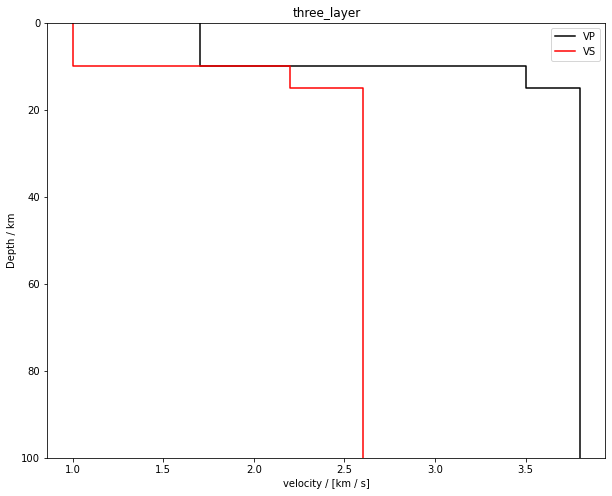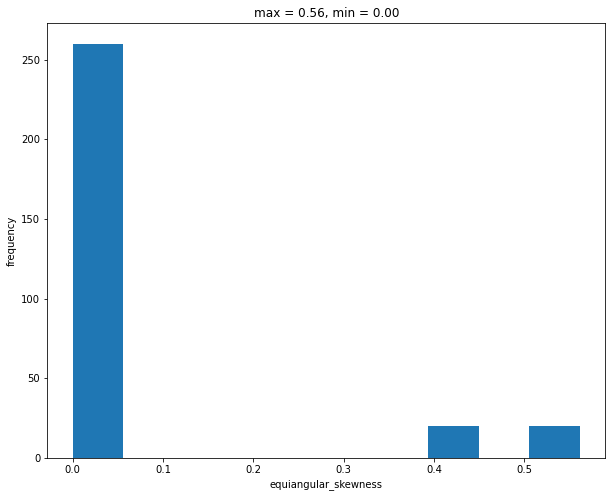Version:

This documentation is not for the latest stable Salvus version.This tutorial is presented as Python code running inside a Jupyter Notebook, the recommended way to use Salvus. To run it yourself you can copy/type each individual cell or directly download the full notebook, including all required files.# Mesh Quality

## Introduction

In this notebook, you build a piecewise structured mesh using a 1D model read from a file and automatic placement of refinements. Play with the input parameters to find out:

• Does this change for 3D?
• Does it depend on the frequency?

The automatic placement considers a number of criteria. If any of them is not met, the refinement is pushed further downwards. This is based on the assumption, that velocities increase with depth in most models (which we enforce by making the size function monotonous before calculating element sizes). The criteria are:

• mimimum resolution in horizontal direction
• no refinement directly at the surface or the bottom
• no multiple refinements at the same depth
• no refinement in very thin layers
Copy
# set up the notebook
%matplotlib inline

import matplotlib.pyplot as plt
import numpy as np

plt.rcParams["figure.figsize"] = (10, 8)

## Building the mesh

The model file, edit as you like with colums in units of km, km/s and kg/m^3.

%%writefile three_layer.bm
NAME         three_layer
UNITS        km
COLUMNS      depth rho vp vs
0.0   2.6     1.7     1.0
10.0   2.6     1.7     1.0
10.0   3.0     3.5     2.2
15.0   3.0     3.5     2.2
15.0   3.5     3.8     2.6
100.0   3.5     3.8     2.6
Writing three_layer.bm


Read the model file and plot the seismic velocities.

from salvus.mesh.models_1D import model

model.plot_vp_vs_profile(depth=True)The model provides the discontinuities and functionality to compute element sizes according to the resolution criterion. Internally, we work with normalized coordinates, hence the need to scale.

print(
"discontinuities:",
["{:.1f}".format(i) for i in model.discontinuities * model.scale],
)
print(
"element size:   ",
[
"{:.1f}".format(i)
for i in model.get_edgelengths(
dominant_period=1.0, elements_per_wavelength=2
)
* model.scale
],
)
discontinuities: ['0.0', '85000.0', '90000.0', '100000.0']
element size:    ['1300.0', '1100.0', '500.0']

##### Exercise: Vary hmax_refinement, refinement_style and refinement_top_down to make the best mesh.

Note: Top down approach means minimizing number of elements at the surface at the cost of more elements at the bottom (default). If False, bottom up approach is used, that is minimizing number of elements at the bottom at the cost of more elements at the surface. Top down leads to fewer refinement. Which one is more efficient depends on the velocity model and refinement style.

frequency = 0.1  # maximum frequency in Hz
max_x = 200000.0  # Domain size in horizontal direction in m
hmax_refinement = (
1.5  # critertion to avoid refinements in thin layers, need to be > 1.0,
)
# default is 1.5, smaller value = more aggressive
refinement_style = "doubling"  # 'doubling' or 'tripling'
refinement_top_down = True  # True or False
ndim = 2  # 2 or 3
from salvus.mesh.skeleton import Skeleton

if ndim == 2:
horizontal_boundaries = (np.array(), np.array([max_x / model.scale]))
elif ndim == 3:
horizontal_boundaries = (
np.array([0, 0]),
np.array([max_x / model.scale, max_x / model.scale]),
)

sk = Skeleton.create_cartesian_mesh(
model.discontinuities,
model.get_edgelengths(1.0 / frequency),
hmax_refinement=hmax_refinement,
horizontal_boundaries=horizontal_boundaries,
refinement_top_down=refinement_top_down,
refinement_style=refinement_style,
ndim=ndim,
)
m = sk.get_unstructured_mesh()
m.find_side_sets(mode="cartesian")
m
When run interactively in a Jupyter Notebook, this output cell contains a widget. Click the button below to load a preview of it. Please note that most interactive functionality does not work without a running Python kernel.
<salvus.mesh.unstructured_mesh.UnstructuredMesh at 0x7fcdd62dd1d0>

## Judging the quality

#### a) Equiangular Skewness

A popular quality measure in the community is the equiangular skewness, which is defined as

\begin{align} \text{skew} = \max \left( \frac{\theta{\max} - \theta{e}}{180 - \theta{e}}, \frac{\theta{e} - \theta{\min}}{\theta{e}} \right). \end{align}

Quality meshes must not have skewed elements (skewness <~ 0.75), a single bad element can cause instability in the time extrapolation.

m.plot_quality("equiangular_skewness")Locate skewed elements visually:

m.attach_field(
"equiangular_skewness", m.compute_mesh_quality("equiangular_skewness")
)
m
When run interactively in a Jupyter Notebook, this output cell contains a widget. Click the button below to load a preview of it. Please note that most interactive functionality does not work without a running Python kernel.
<salvus.mesh.unstructured_mesh.UnstructuredMesh at 0x7fcdd62dd1d0>

#### b) resolution criterion

Another important quality criterion is the resolution of the waves at the specified frequency, that is the elements need to be smaller than a constant times the local wavelength:

\begin{align} h{\max} < \frac{\lambda}{n} = \frac{v{s}}{f n}, \end{align}

where $f$ is the frequency, $h_{\max}$ is the longest edge of the element an $n$ is the number of elements used per wavelength (typically 2). This criterion is not strict in the sense that it is no problem if it is violated by a few elements.

As this was an input to the mesh generation routine, we should expect that this criterion is fulfilled here.

hmax = model.get_edgelengths_radius(
m.get_element_centroid()[:, -1], dominant_period=1.0 / frequency
)

h = m._hmax() / hmax
print("h_min = {0}, h_max = {1}".format(h.min(), h.max()))
m.attach_field("h", h)

m
h_min = 0.45454545454545486, h_max = 1.0000000000000009

When run interactively in a Jupyter Notebook, this output cell contains a widget. Click the button below to load a preview of it. Please note that most interactive functionality does not work without a running Python kernel.
<salvus.mesh.unstructured_mesh.UnstructuredMesh at 0x7fcdd62dd1d0>

#### c) Simulation Cost

We can estimate the simulation cost to be proportional to number of elements / time step to compare different meshes. The largest stable time step in explicit time stepping schemes can be estimated based on the Courant criterion:

\begin{align} C = \frac{v{p} \Delta t}{h{\min}} < C_{\max}, \end{align}

where $h_{\min}$ is the minimum point distance in each element, $\Delta t$ the time step and $C$ the Courant number. $C_{\max}$ depends on the time scheme and a typical value is $0.4$.

While the $h_{\min}$ is often just computed based on the edgelengths of each element, salvus has a more accurate estimator that takes the deformation of the elements into account. With this more accurate cost estimate, one may find even more skewed elements acceptable, as long as they do not result in an unfeasible time step.

Note that the $\Delta t$ estimated here needs to be scaled by the courant number and GLL point distance for the order of the spectral elements to get the final time step.

z = m.get_element_centroid()[:, -1]
vp = model.get_elastic_parameter("VP", z, scaled=False)

# edgelength based estimate
dt1, dt1_elem = m.compute_dt(vp, fast=True)

# more accurate estimate
dt2, dt2_elem = m.compute_dt(vp, fast=False)

print("number of elements:   %i" % m.nelem)
print("edgelength based dt:  %.2f" % dt1)
print("accurate dt:          %.2f" % dt2)
print("cost factor:          %.1f" % (m.nelem / dt2))
number of elements:   300
edgelength based dt:  1.32
accurate dt:          0.61
cost factor:          493.1


plot dt over the mesh to locate the minimum:

m.attach_field("dt1", dt1_elem)
m.attach_field("dt2", dt2_elem)
m
When run interactively in a Jupyter Notebook, this output cell contains a widget. Click the button below to load a preview of it. Please note that most interactive functionality does not work without a running Python kernel.
<salvus.mesh.unstructured_mesh.UnstructuredMesh at 0x7fcdd62dd1d0>PAGE CONTENTS